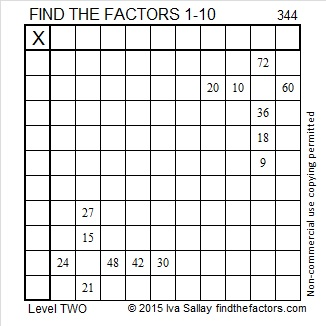# 344 and Level 2

Quick Prime or Composite Number Test: 344 is even and thus divisible by 2, so 344 is a composite number.Print the puzzles or type the factors on this excel file: 10 Factors 2015-01-05

• 344 is a composite number.
• Prime factorization: 344 = 2 x 2 x 2 x 43, which can be written (2^3) x 43
• The exponents in the prime factorization are 3 and 1. Adding one to each and multiplying we get (3 + 1)(1 + 1) = 4 x 2 = 8. Therefore 344 has exactly 8 factors.
• Factors of 344: 1, 2, 4, 8, 43, 86, 172, 344
• Factor pairs: 344 = 1 x 344, 2 x 172, 4 x 86, or 8 x 43
• Taking the factor pair with the largest square number factor, we get √344 = (√4)(√86) = 2√86 ≈ 18.547This site uses Akismet to reduce spam. Learn how your comment data is processed.